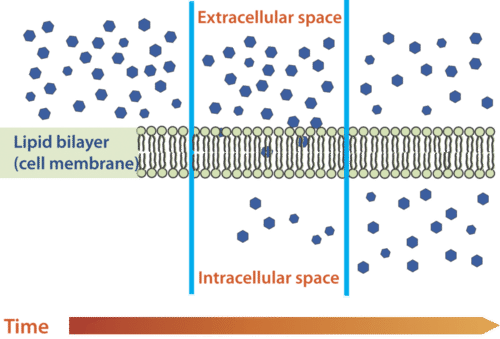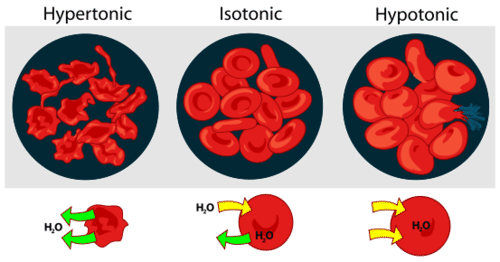# 2.1: Diffusion

$$\newcommand{\vecs}{\overset { \rightharpoonup} {\mathbf{#1}} }$$ $$\newcommand{\vecd}{\overset{-\!-\!\rightharpoonup}{\vphantom{a}\smash {#1}}}$$$$\newcommand{\id}{\mathrm{id}}$$ $$\newcommand{\Span}{\mathrm{span}}$$ $$\newcommand{\kernel}{\mathrm{null}\,}$$ $$\newcommand{\range}{\mathrm{range}\,}$$ $$\newcommand{\RealPart}{\mathrm{Re}}$$ $$\newcommand{\ImaginaryPart}{\mathrm{Im}}$$ $$\newcommand{\Argument}{\mathrm{Arg}}$$ $$\newcommand{\norm}{\| #1 \|}$$ $$\newcommand{\inner}{\langle #1, #2 \rangle}$$ $$\newcommand{\Span}{\mathrm{span}}$$ $$\newcommand{\id}{\mathrm{id}}$$ $$\newcommand{\Span}{\mathrm{span}}$$ $$\newcommand{\kernel}{\mathrm{null}\,}$$ $$\newcommand{\range}{\mathrm{range}\,}$$ $$\newcommand{\RealPart}{\mathrm{Re}}$$ $$\newcommand{\ImaginaryPart}{\mathrm{Im}}$$ $$\newcommand{\Argument}{\mathrm{Arg}}$$ $$\newcommand{\norm}{\| #1 \|}$$ $$\newcommand{\inner}{\langle #1, #2 \rangle}$$ $$\newcommand{\Span}{\mathrm{span}}$$$$\newcommand{\AA}{\unicode[.8,0]{x212B}}$$

### What happens if you put a few drops of food coloring in water?

Over time, the molecules of color spread out through the rest of the water. When the molecules are evenly spread throughout the space, the water will become an even color. This process of molecules moving from an area where there are lots of molecules to an area where there are fewer molecules is known as diffusion.

## Diffusion

Small molecules can pass through the plasma membrane through a process called diffusion. Diffusion is the movement of molecules from an area where there is a higher concentration (larger amount) of the substance to an area where there is a lower concentration (lower amount) of the substance (Figure below). The amount of a substance in relation to the total volume is the concentration. During diffusion, molecules are said to flow down their concentration gradient, flowing from an area of high concentration to an area of low concentration. Molecules flowing down a concentration gradient is a natural process and does not require energy.

Diffusion can occur across a semipermeable membrane, such as the cell membrane, as long as a concentration gradient exists. Molecules will continue to flow in this manner until equilibrium is reached. At equilibrium, there is no longer an area of high concentration or low concentration, and molecules flow equally in both directions across the semipermeable membrane. At equilibrium, equal amounts of a molecule are entering and leaving a cell.Figure $$\PageIndex{1}$$: Diffusion is the movement of a substance from an area of a higher amount toward an area of lower amount. A concentration gradient initially exists across the cell membrane. Equilibrium is reached when there is an equal amount of the substance on both sides of the membrane.

### Osmosis

The diffusion of water across a membrane because of a difference in concentration is called osmosis. Let's explore three different situations and analyze the flow of water.

1. A hypotonic solution means the environment outside of the cell has a lower concentration of dissolved material than the inside of the cell. If a cell is placed in a hypotonic solution, water will move into the cell. This causes the cell to swell, and it may even burst.
2. A hypertonic solution means the environment outside of the cell has more dissolved material than inside of the cell. If a cell is placed in a hypertonic solution, water will leave the cell. This can cause a cell to shrink and shrivel.
3. An isotonic solution is a solution in which the amount of dissolved material is equal both inside and outside of the cell. Water still flows in both directions, but an equal amount enters and leaves the cell.

### Applications of Osmosis

How do marine animals keep their cells from shrinking? How do you keep your blood cells from bursting? Both of these questions have to do with the cell membrane and osmosis. Marine animals live in salt water, which is a hypertonic environment; there is more salt in the water than in their cells. To prevent losing too much water from their bodies, these animals intake large quantities of salt water and then secrete the excess salt. Red blood cells can be kept from bursting or shriveling if put in a solution that is isotonic to the blood cells. If the blood cells were put in pure water, the solution would be hypotonic to the blood cells, so water would enter the blood cells, and they would swell and burst (Figure below).Figure $$\PageIndex{2}$$: Osmosis causes these red blood cells to change shape by losing or gaining water.

## Summary

• Diffusion is the movement of molecules from an area of high concentration to an area of low concentration.
• The diffusion of water across a membrane because of a difference in concentration is called osmosis.

## Explore More

Use the resource below to answer the following questions.

1. What is osmosis?
2. What is tonicity?
3. How can a hypotonic solution cause a cell to rupture? Describe this process as specifically as you can.
4. How would a hypertonic solution affect a cell? How could this affect cellular processes?
5. Do water molecules leave or enter a cell in an isotonic solution?

Review

1. Describe the process of diffusion.
2. Define osmosis.
3. If a plant cell is placed in a solution and the cell shrivels up, what type of solution was it placed in? How do you know?

2.1: Diffusion is shared under a CC BY-NC license and was authored, remixed, and/or curated by LibreTexts.